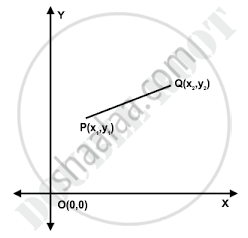# Distance Formula

## Notes

Here what will be the distance between points PQ? To find this we will use distance formula.Distance formula says, PQ= sqrt[ (x_2-x_1)^2 + (y_2-y_1)^2]
Example1- Find the distance between the points P(6,-6) and O(0,0)
Solution: x_2=0, x_1=6, y_2=0 and y_1=-6
PO= sqrt[ (x_2-x_1)^2 + (y_2-y_1)^2]
= sqrt[ (0-6)^2 + (0+6)^2]
= sqrt[ (-6)^2 + (6)^2]
= sqrt[ 36+36]
= sqrt72
PO = 6 sqrt2 units
Example2- Find the values of x for which the distance between the points P(4,-5) and Q(12,x) is 10 units
Solution: PQ=10
x_2= 12, x_1=4, y_2=x and y_1=-5
PQ= sqrt[ (x_2-x_1)^2 + (y_2-y_1)^2]
10  = sqrt[ (12-4)^2 + (x+5)^2]
= sqrt[ (8)^2 + (x+5)^2]
= sqrt[ 64 + (x+5)^2]
Sqauring both the sides
100= 64+ (x+5)^2
36= (x+5)^2
+or-6 = x+5
x= 6-5 or x= -6-5
x=1 or x= -11
Example3- Find the point P on x-axis which is equidistance from the points A(5,-2) and B(-3,2)
Solution: Let the point be P(x,0)
Now, PA=PB [Given as point P on x-axis is equidistance from the points A(5,-2) and B(-3,2)]
"PA"^2="PB"^2
(x_2-x_1)^2 + (y_2-y_1)^2 = (x_2-x_1)^2 + (y_2-y_1)^2
(5-x)^2 + (-2-0)^2 = (-3-x)^2 + (2-0)^2
25+x^2-10x+4 = (-3)^2 +x^2 -2(-3)(x) +4
25+x^2-10x+4 = 9+x^2+6x+4
16x= 16
x=1
Therefore, point P is (1,0)

If you would like to contribute notes or other learning material, please submit them using the button below.

### Shaalaa.com

Coordinate Geometry part 2 (Distance Formula) [00:10:15]
S